# Continuous operator

A continuous mappingof a subsetof a topological space, which as a role is also a vector space, into a spaceof the same type, specifically: A mapping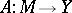,, is continuous at a pointif for any neighbourhood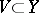of the point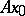there is a neighbourhood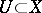ofsuch that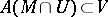; a mappingis continuous on a setif it is continuous at every point of.

For an operatorto be continuous onit is necessary and sufficient that for every open (closed) setthe complete inverse image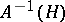is the trace onof an open (closed) set in, that is,, whereis open (closed) in. For continuous operators the chain rule holds: Suppose that,, is continuous on(or at) and suppose also that,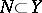, is continuous on(or at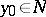). If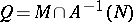is not empty (or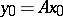), then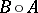is continuous on(or at).

Whenandare topological vector spaces andis a linear continuous operator on a linear subspace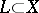with values in, then the continuity ofat some point of, say, at the origin, implies the continuity ofon the whole of. A continuous operator on a submanifoldof a topological vector spaceis bounded on, that is, the image of any bounded set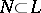is bounded in. Ifandare separable, then the compactness ofimplies that of.

An operatoris uniformly continuous onif for any neighbourhood of the originthere exists a neighbourhood of the originsuch thatimplies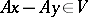. An operator that is linear and continuous on a linear submanifold of a topological vector space is automatically uniformly continuous on this submanifold.

Apart from continuity one introduces the concept of countable continuity of an operator. An operatoris countably continuous atif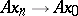for any sequence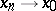,. For metrizable spaces continuity and countable continuity coincide.

How to Cite This Entry:
Continuous operator. V.I. Sobolev (originator), Encyclopedia of Mathematics. URL: http://encyclopediaofmath.org/index.php?title=Continuous_operator&oldid=14154
This text originally appeared in Encyclopedia of Mathematics - ISBN 1402006098